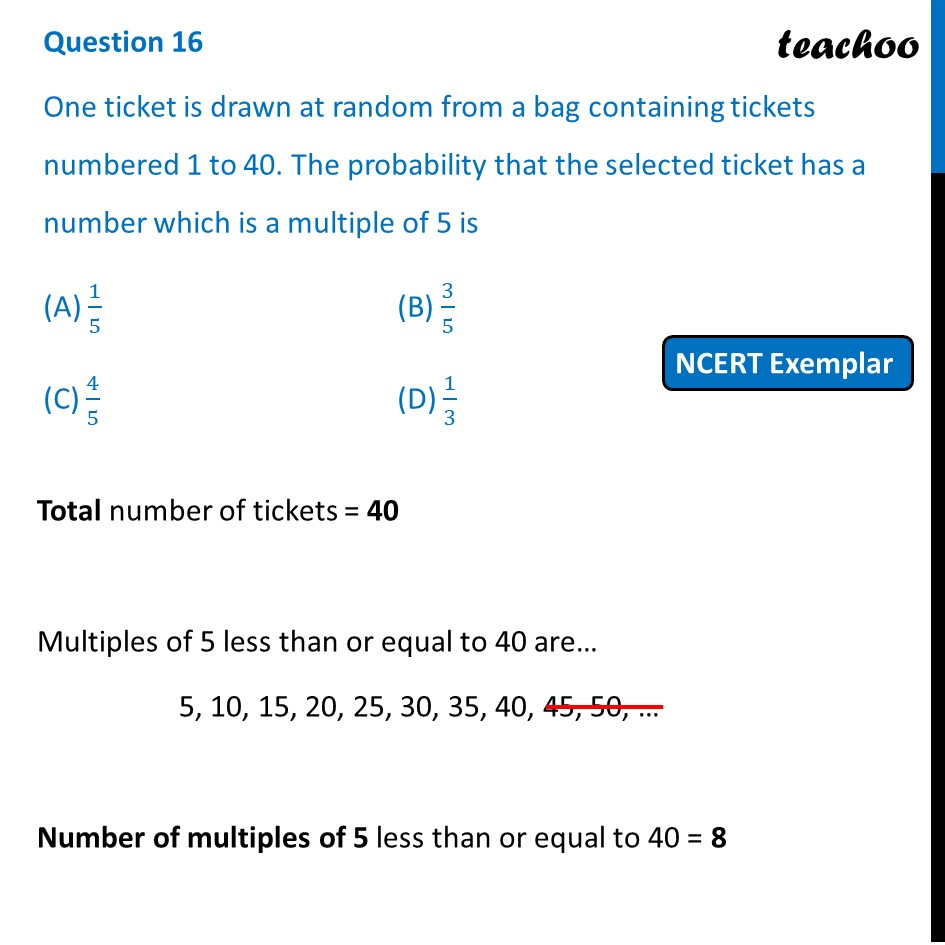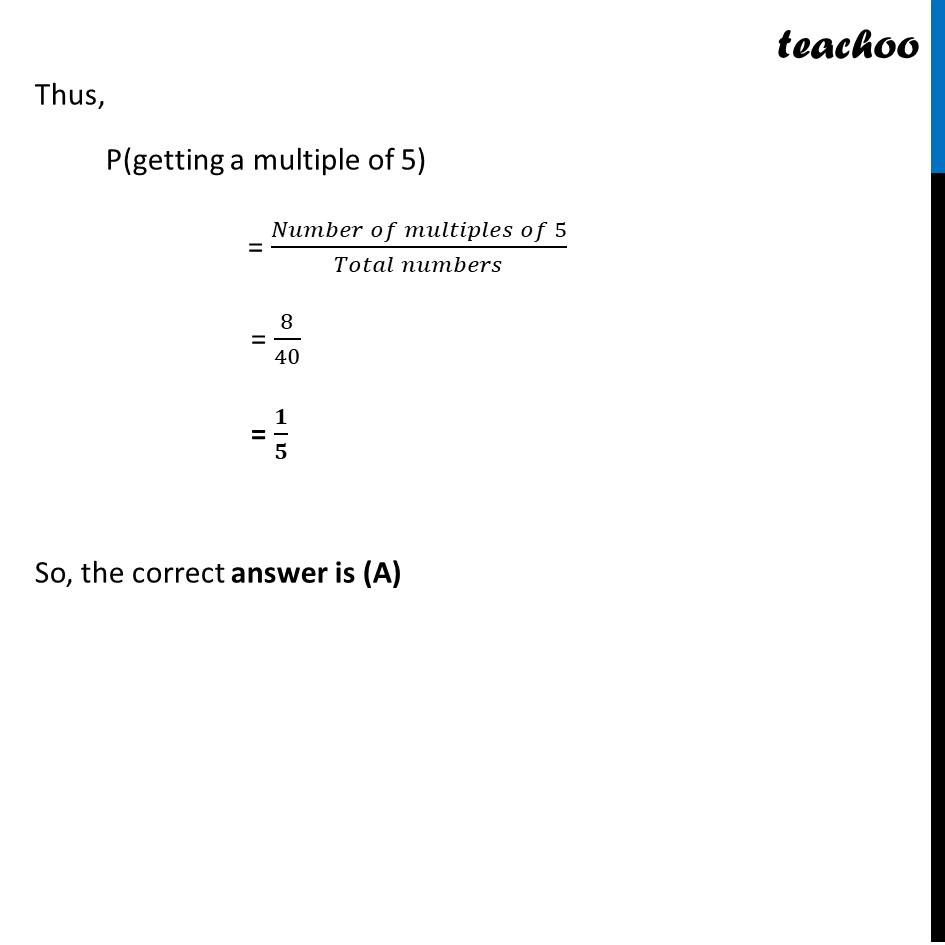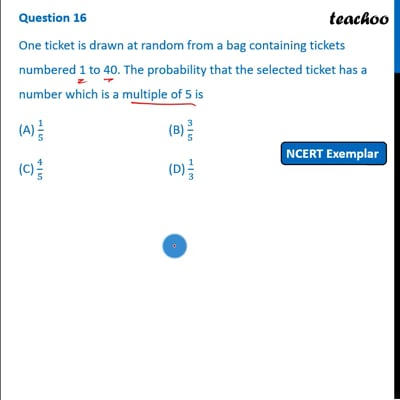NCERT Exemplar - MCQ

Chapter 15 Class 10 Probability
Serial order wise

## (C) 4/5   (D) 1/3This video is only available for Teachoo black users

Get live Maths 1-on-1 Classs - Class 6 to 12

### Transcript

Question 16 One ticket is drawn at random from a bag containing tickets numbered 1 to 40. The probability that the selected ticket has a number which is a multiple of 5 is (A) 1/5 (B) 3/5 (C) 4/5 (D) 1/3 Total number of tickets = 40 Multiples of 5 less than or equal to 40 are… 5, 10, 15, 20, 25, 30, 35, 40, 45, 50, … Number of multiples of 5 less than or equal to 40 = 8 Thus, P(getting a multiple of 5) = (𝑁𝑢𝑚𝑏𝑒𝑟 𝑜𝑓 𝑚𝑢𝑙𝑡𝑖𝑝𝑙𝑒𝑠 𝑜𝑓 5)/(𝑇𝑜𝑡𝑎𝑙 𝑛𝑢𝑚𝑏𝑒𝑟𝑠) = 8/40 = 𝟏/𝟓 So, the correct answer is (A)